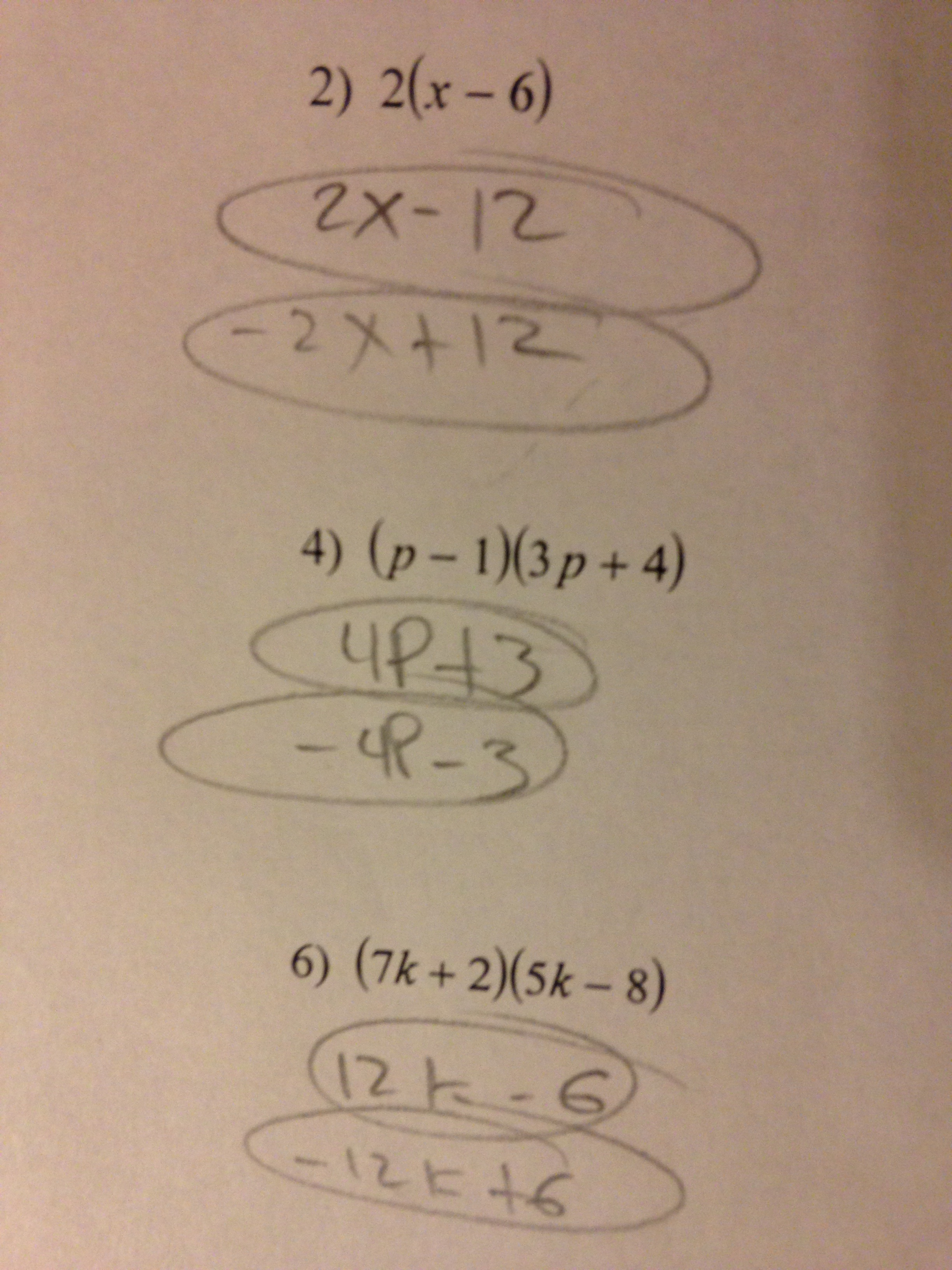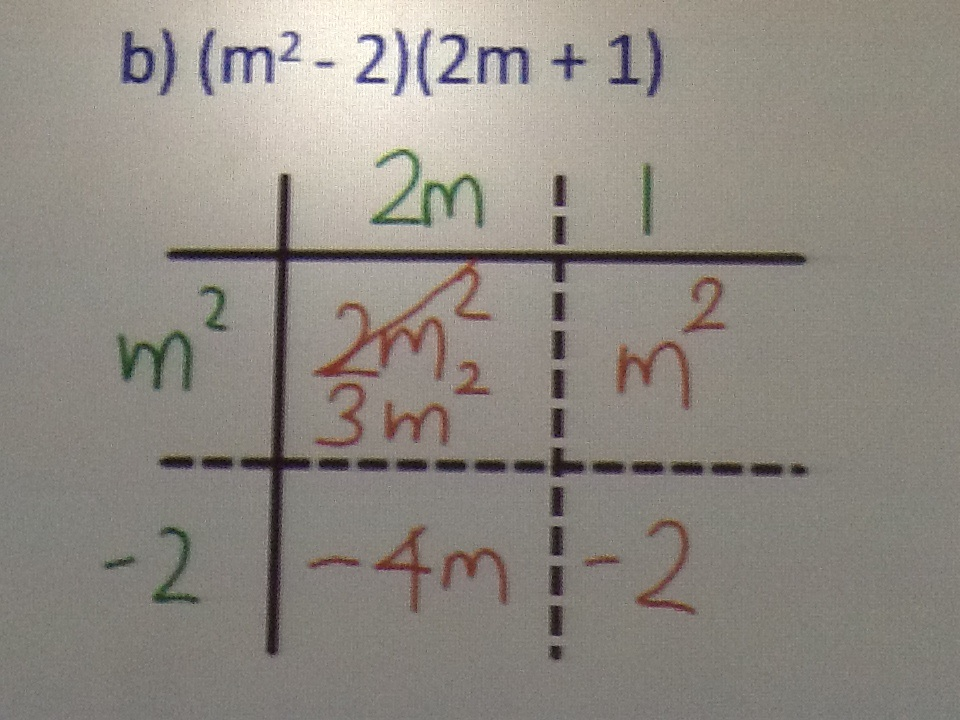Categories

## Inconsistent Polynomial MultiplicationWhy does this student answer$2a + 9$ for the first question, and$x + 49$ for the second questions?

Why does this student express more confidence on the first question than the second question?

This post is part of a series analyzing a bunch of survey results. For previous posts, go here, here and here.

Categories

## Diving into the Multiplying Polynomials SurveyThis is from yesterday’s survey, which was discussed over at this post. What do you make of the responses, particularly the differences between  (2a+6) in the first response, and (2x+49) in the second?

This post is part of a series analyzing a bunch of survey results. For previous posts, go here and here.

Categories

## Another pre-instruction survey, this time about polynomial multiplication

View this document on Scribd

Noteworthy:

• The kids have a ton of confidence, even in the stuff that they haven’t formally studied in class yet.  (For this survey, Questions 1-3 had been covered formally, and Questions 4-5 had not.) To my mind, this continues to reaffirm that the most annoying mistakes aren’t the distortion of instruction; they’re the failure of instruction to override preconceptions.
• Kids like to say that$(x+7)^2 = 49$, and teachers like to say that this is due to overuse of the Distributive Property. That might be true, but those teachers also have to recognize that kids said that$(a+3)(a+3)=a^2 + 9$ with almost the same verve and frequency. It’s hard to blame exponents or notation for that mistake, right? So where does this intuition come from?
• A couple of kids included a$2a$ term in Q4 and a$x^2$ term in Q5. I find this interesting, but I’m not exactly sure what its significance is. Is the temptation to add$a+3$ and$a+3$ when the binomials are in the same visual position that they are for addition problems?

The idea that kids walk into our classes with these intuitions is, I think, counter to the way that most math teachers talk and think about these mistakes. I think that realizing that these mistakes are the result of deep intuitions about how math should be is important. I also think thinking about where these intuitions come from is important, because maybe we can avoid setting them in earlier years.

I hope that some of you will give this survey to your students who haven’t yet received instruction on how to multiply polynomials. The original survey can be found here.

You’ll disagree with me in the comments, right? I’m counting on you all…

Categories

## Multiplying monomials and binomialsThere’s a lot going on here. What about this work do you find the most interesting? How would you help this student?

Thanks to Heather for the submission!

Categories

## Multiplying Monomials and BinomialsWhy would$2m \times m^{2} = 3m^{2}$? Or$2m^{2}$?

This mistake was brought to you by the letters M, A, R, Y, B, O, U, R, A, S, S, and A. You can follow her on twitter and on her blog.